Circuits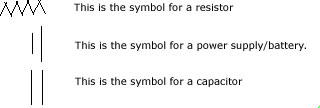When resistances are connected in series, the sum of the resistances in the circuit will equal the equivalent resistance(the resistance that would be there if there were only one resistor) R_eq = R_1 + R_2 +.. etc. The sum of the voltage V1+V2+V3.. equals the total voltage V. Since this is true, then V = IR_eq. When in parallel, the reciprrocal of the total resistance equals the sum of the reciprocols of the individual resistances.. 1/R_eq = 1/R_1 + 1/R_2, etc. I, the total current is the sum of the partial currents. I = I_1 + I_2 + I_3.. etc. I = V/R_eq. ACCORDING TO RETROPUNK, if you have two parallel resistors, R1, R2, then the equivalent resistance is R1*R2/(R1+R2)

Finding net capacitance is just the opposite. When in parallel, the sum of the individual capacitances equals the net capacitance. When in series, 1/net capacitance would equal 1/C1 + 1/C2 +1/C3.. and so on.

Terminal voltage from point a to point b = electromotiveforce - Ir. the internal resistance is r, I is current, electromotive force is the potential difference between the terminals of a source like a battery when no current flows to an external circuit, or in other words when no other device is connected.

The sum of the potential difference around a closed circuit is 0. ( LOOP RULE, Kirchoff ) The sum of the current entering a junction and the current leaving a junction is equal to 0. ( JUNCTION RULE, Kirchoff )

 The cube made of resistors.. Sorry, no picture! Having a cube of wires, each wire acting as a R-ohm resistor, and the cube is connected to the circuit through it's diagonals, that is, two opposite corners. Find the equivalent resistance. This problem seems rather complex, but by spending some time analyzing the situation, you can break down this cube of resistors into a simple circuit. Starting at one corner, the current will split into three parallel resistors. Every wire that has the same potential can be "connected." This is because no current will flow through the connection. We then have the first part of the cube, three resistors in parallel. The next step is to bridge the mass of resistors to get back to the other corner via 3 more parallel resistors ( symmetry of the cube ). There are six resistors left in the middle. They are actually in parallel with each other if you draw out the cube as a flattened cube ( try it! ). Putting it all together, we arrive at (3(1/R))^-1 + (6(1/R))^-1 + (3(1/R))^-1 => (5/6)*R Wheatstone Bridge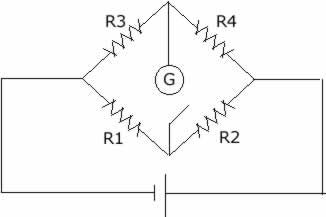This is a circuit used to make precise measurements of resistance. Generally speaking R4 is the unknown resistance. Try deriving the following formula! "R4*R1 = R2*R3" Voltage through arm 2 is equal to voltage to arm 4., voltage through arm 1 is equal to voltage through arm 3. The voltage through the galvanometer is 0, no current through the galvanometer either. From this we can deduce that I1 = I2, and I3 = I4. V2 = I2R2. V4 = I4R4. Since V4 = V2, then I2R2 = I4R4 is true. The total voltage equals V2 + V1 and also equals V3 + V4. Since V2 = V4, then V4 + V1 = V3 + V4, V1 = V3. V1 = I1R1, V3 = I3R3. From above, I1 = I2, I3 = I4. V1 = V3. I1R1 = I3R3 I2R1 = I4R3, from above I2R2 = I4R4, divide the two. R1/R2 = R3/R4. R4R1/R2 = R3. R4 = R2R3/R1. The T-Circuit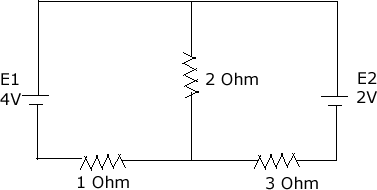This is the T-Circuit. Try to solve for the current in each branch! BECAUSE PHYSICS IS FUN AND EVERYONE LOVES PHYSICS!!@% I2 = I1 + I3 JUNCTION E1 = I2R2 + I1R1 LOOP E1 + I3R3 = E2 + I1R1 LOOP Since I2 = I1+I3, then eq 2 can be rewritten as E1 = I1R2 + I3R2 + I1R1. (Thanks for correction Jaia) Also, retropunk's suggested node voltage method: (V(2ohm) - E1) + V(2ohm)/2 + (V(2ohm) - E2)/3 =0

RC Circuits
Resistor capacitor circuits are a special kind of circuit. Here we begin to dive back into charges and the such.
tau = time constant = RC, measures how quickly a capacitor charges.
half-life(the time it takes to charge the capacitor to half full)= tau * ln 2

Capacitor Charging formulas.
V = emf(1-e^-t/RC)
q = q_0(1-e^-t/RC) , q is charge, q_0 is max charge.

Capacitor Discharging formula
V = V_0(e^-t/RC), V_0 being the initial voltage.

Problem 1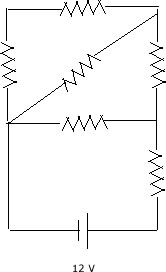In the diagram to the left, each of the resistors have a resistance of 2 ohms. Find the equivalent resistance throughout the circuit. To tackle circuit problems, basically all you want to do is look where you can start simplifying. For starters, the top left and top center resistances can be added together to get 2R since they are in series. Next you can simplify again by taking the recipricol of that and adding the recipricol of R, the diagonal resistance. Next, it acts as in series with the resistor in the top right. so add that. Here is what you should have so far.. (2R^-1 + R^-1)^-1 +R. Next, is the middle horizontal resistor, which is in parallel with what we have so far.. So we now have. (((2R^-1 + R^-1)^-1 +R)^-1 + R^-1)^-1. And to finish it off, we add the resistor closest to the power source as a series. (((2R^-1 + R^-1)^-1 +R)^-1 + R^-1)^-1 + R. Yeah.. it's a little messy but breaking it down will help. You can also redraw the diagram as a circuit getting rid of the diagonal resistor. So, just substitute 2 ohms in for R, and you will get 3.25 ohms.

Problem 2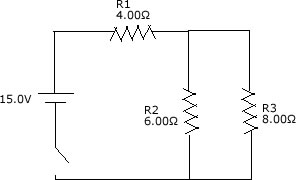Find I1, I2, I3, V1, V2, V3 and the power loss in R3. Start off by finding the equivalent resistance, R1+ (R2^-1 +R3^-1)^-1 => 7.4 ohms. Now, use the equation V_powersupply = I_total*R_eq. 15/7.4 = 2.027A. R1 will receive the full current since it isn't in parallel with anything and has a series connection to the power supply. So, I1 = 2.027A. Solve for V1 by multiplying by R, 2.027*4.00 = 8.1V. Use the loop rule now, V_powersupply - V1 = V2 = V3. So, 15.0V - 8.1 = 6.9V for both V2 and V3. Now you can apply Ohm's law, and find the current to I2 is 1.15A, and I3 is 0.8625A. The power loss in R3 is simply P = I^2R(or P = IV), so P = (0.8625^2)(8.00) = 5.95W.

Problem 3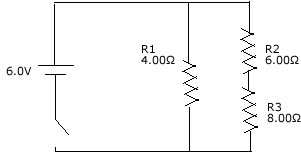Find I1, I2, I3, V1, V2, V3 and the power loss in R3. Find the equivalent resistance of the circuit.. Should be (R1^-1 + (R2+R3)^-1)^-1. This will equate 3 ohms. Now that you have the equivalent resistance, V_powersupply = I_total*R_eq, 6.0V/3.0 ohms = 2.0 Amps for total current. Use the loop rule to determine that V_powersupply = V1, so V1= 6.0V. Now solve for I1, 6.0V/6.0 ohms= 1.0A. Since we found the current to this branch of the junction, then the total minus this current must be the current for the branch that R2 and R3 resides on. I_total - I1 = 1.0A. Thus I2 and I3 are 1.0A since they are in series. Now, solve for V2 and V3 by Ohm's law, giving 4V and 2V respectively.

Problem 4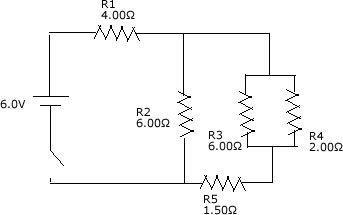Find I1, I2, I3, I4, I5, V1, V2, V3, V4, V5 and the power loss in R. Find the equivalent resistance of the circuit. R1 + (R2^-1 + ((R3^-1+R4^-1)^-1+R5)^-1 = 6 ohms. V_powersupply = I_total*R_eq, 6.0V/6.0 ohms = 1A for total current. R1 receives the full current since it is in series, I1 = 1A. Solve for V1 by Ohm's law; 4.0V. Use the loop rule to find V2 now, V_powersupply - V1 = V2, 6.0-4.0 = 2.0V. Use Ohm's law again to find I2, which is 0.33A. Now, find the current for the branch containing R3, R4 and R5. Since I2 is 0.33A, and the total current is 1.0A, the current entering that branch must be the difference, 0.66A. Since R5 is in series with that current, I5 = 0.66A, use V = IR to find V5 to be 1V. Since V5 is 1, then the loop rule says V_powersupply - V1 - R5 = (V3+V4). 6 - 4 - 1 = 1V, so the voltage is 1V for V3 and V4 because the voltage through parallel resistors is equivalent. Now use Ohm's law again to solve for the currents. I3 = 0.1667A, I4 = 0.5A

Problem 5 (kudos paul)
A 2.8 kilo-ohm and 2.1 kilo-ohm resistor are connected in parallel this combination is connected in series with a 1.8 kilo-ohm resistor. If each resistor is rated at 1/2W, what is the maximum voltage that can be applied across the whole network?

Since this problem is querying for the max voltage, this means that the answer will depend on the resistors. Each resistor having a rating of 1/2W means that only 1/2W is allowed, or else it will blow up like an overcooked chicken (note: not a bad thing to do!) HAha.. anyway. The equivalent resistance for just the parallels would be ((2.8x10^3)^-1 + (2.1x10^3)^-1)^-1 => 1200 ohms. The total equivalent resistance would be 3000 ohms. Let R1 be the 1800 ohm resistor, R2 the 2100 ohm resistor, R3 the 2800 ohm resistor.

For resistor 1, P = V^2/R. Although we know P and R, we need to find a way to express the applied voltage. You know the current will split at the parallel branch, and then the sum of the current here will equal the current running to the 1.8 kilo-ohm resistor. Let's consider the 1.8 kilo-ohm resistor first. If a current is running through the resistor, then voltage can be found via V = IR. R is 1800ohm. If the equivalent resistance is 3000ohm, then the current can also be expressed aby V_powersource = I*3000. As you can see, these are in a ratio of sorts. 1800/3000 is used in expressing V_max. From P = V^2/R, 1/2W = (1800/3000V)^2/(1800) 1800 being the resistance of the resistor, solving for V will result in 50V or -50V.

For resistor 2, P = V^2/R. Just like for resistor 1, we have generalized how to approach this. Keeping in mind that the R divided by the equivalent resistance will be the R that the current encounters when traveling to a parallel branch. So, use the 1200ohm. 1/2W= (1200/3000V)^2/2100 => 81V or -81V.
For resistor 3, P = V^2/R, repeat.. 1/2W = (1200/3000V)^2/2800 => 93.54V or -93.54V

Looks like 50V is the lowest voltage, so 50V is the maximum voltage that can be applied across the whole network.

Problem 6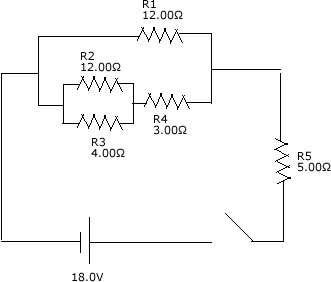You know the deal.. Find I1, I2, I3, I4, I5, V1, V2, V3, V4, V5 and the power loss in R3. R_eq should be (((R2^-1+r3^-1)+R4)^-1+R1^-1)^-1 +R5, which equates to 9.00 ohms. Find I_total by V = IR, 18/9 = 2 amps. R5 is the only resistor that will get the full current of 2 amps, so I5 = 2A, V5 = 10. Now that we have found the potential difference for R5, we can find the potential difference running through the parallel branch by the loop rule. V_source - V5 = Par branch. 18V - 10V = 8V goes to the branch. Since R1 is not in series with another circuit, it will get the full 8V, so V1 = 8V, I1 = 0.6667 amps. Use the junction rule to find the current running through the lower branch containing R2 R3 and R4, I_total - I1 = current through branch, 2 - 0.6667 = 1.333 amps. R4 is not in parallel so it will receive that full 1.333 amps, I4 = 1.333 amps. V4 = 3.999V. Now we are left with R2 and R3. By the loop rule, V1 - V4 = V2 = V3(since R2 and R3 are in parallel they receive the same voltage). So, 8 - 3.999...= 4V. Now that you have V2 and V3 equal to 4V, solve for I2 and I3, which are 0.333 amps and 1 amp respectively. To find the power loss in R3, use P=I^2R, which will give you 4W.

Problem 7
An unknown length of platinum wire, 0.92 mm in diameter is placed as the unknown resistance in a Wheatstone bridge(see top). R1 is 38 ohms, R2 is 46 ohms, Balanced is achieved when R3 is 3.48 ohms. How long is the platinum wire?

Remember R = pL/A from the Electric Currents section? Well, that'll definitely help here. p for platinum is 10.6 x 10^-8 ohm*meters.
So, since we know of the relationship R4R1 = R2R3, this should be a breeze. R4 = (R2R3)/R1
R4 = (46*3.48)/38 = 4.2126 ohms.
Now use R = pL/A.
4.2126 = (10.6x10^-8)L/ pi((1/2*.92 x 10^-3)^2)
(4.2126*pi((1/2*.92 x 10^-3)^2))/ 10.6x10^-8 = L
26.4m = L

Problem 8
If a charged capacitating duck C = 45F is connected to a resistance R = 500 ohms, how much time will elapse until the voltage falls to 10 percent of its original value?

V = V_0(e^-t/RC)
.1V = V_0(e^-t/RC)
.1 = e^-t/(45*500)
ln .1 = -t/(22500)
51808 seconds = t